# Birkhoff-Witt theorem

(Redirected from Birkhoff–Witt theorem)
Jump to: navigation, search

Poincaré–Birkhoff–Witt theorem

A theorem about the representability of Lie algebras in associative algebras. Letbe a Lie algebra over a field, let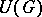be its universal enveloping algebra, and let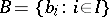be a basis of the algebrawhich is totally ordered in some way. All the possible finite products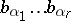, where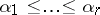, then form a basis of the algebra, and it thus follows that the canonical homomorphismis a monomorphism.

It is possible to construct a Lie algebra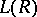for any associative algebra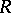by replacing the operation of multiplication inwith the commutator operation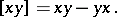The Birkhoff–Witt theorem is sometimes formulated as follows: For any Lie algebraover any fieldthere exists an associative algebraover this field such thatis isomorphically imbeddable in.

The first variant of this theorem was obtained by H. Poincaré ; the theorem was subsequently completely demonstrated by E. Witt  and G.D. Birkhoff . The theorem remains valid ifis a principal ideal domain , in particular for Lie rings without operators, i.e. over, but in the general case of Lie algebras over an arbitrary domain of operators the theorem is not valid .